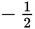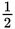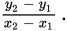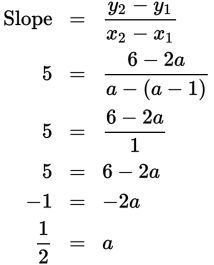# SAT Math Multiple Choice Question 369: Answer and Explanation

### Test Information

Question: 369

9. If a line that passes through the coordinates (a - 1, 2a) and (a, 6) has a slope of 5, what is the value of a?

• A. -2
• B.• C.• D. 2

Explanation:

C

Difficulty: Medium

Category: Heart of Algebra / Linear Equations

Strategic Advice: Given two points (even when the coordinates are variables), the slope of the line isGetting to the Answer: You are given a numerical value for the slope and a pair of coordinate points with variables. To find the value of a, plug the points into the slope formula, and then solve for a.